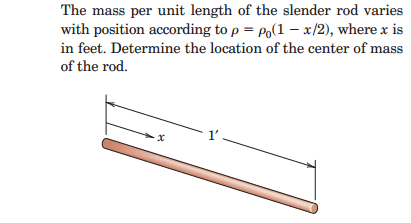# Center of mass of a slender rod with variable density

Hi to everybody

## Homework Statement

I´ll show the problem with a picture:## Homework Equations

L$\overline{x}$=∫xc ρ dl

## The Attempt at a Solution

Well the total lenght of the rod is 1 feet, I only need to calculate the integral.

The moment xc of a differential element of mass of the rod is the distance x to the y axis, and the density is known so:

∫x * (1-x/2) dx , from 0 to 1, the result for me is:

1 * $\overline{x}$ = 1/3

So $\overline{x}$= 1/3;

Unfortunately the result is 4/9, I can´t see where are my mistakes, maybe I´m not using the proper arm lenght or something.

The problem doesn´t give you any coordinate system, only the x axis, I assume that the y axis is orthogonal, and the equation for the moments around that axis gives you the x center of mass.

I suppose that nothing would change if I had used another coordinate system, Am I right?

I assumed too that the constant ρ0 at the equation for the density would not change anything, but maybe that is a mistake.

Where are my mistakes?

Doc Al
Mentor

## Homework Equations

L$\overline{x}$=∫xc ρ dl
Instead of L*xcm, the left hand side should be M*xcm. Where M is the mass of the rod.

I assumed too that the constant ρ0 at the equation for the density would not change anything, but maybe that is a mistake.
You can't just drop a constant, like you did when you integrated.Last edited:
Instead of L*xcm, the left hand side should be M*xcm. Where M is the mass of the rod.

You can't just drop a constant, like you did when you integrated.-Ups, you are right I was used to use the equation of the centroids with constant density and I wrote the wrong equation.

-I see, they cancel after integrating on both parts of the equation.

Now I get the right result.

Thank you very much!!!